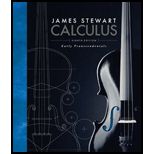# Suppose f is a continuous function defined on a rectangle R = [ a , b ] × [ c , d ]. (a) Write an expression for a double Riemann sum of f. If f ( x , y ) ≥ 0, what does the sum represent? (b) Write the definition of ∬ R f ( x , y ) d A as a limit. (c) What is the geometric interpretation of ∬ R f ( x , y ) d A if f ( x , y ) ≥ 0? What if f takes on both positive and negative values? (d) How do you evaluate ∬ R f ( x , y ) d A ? (e) What does the Midpoint Rule for double integrals say? (f) Write an expression for the average value of f .### Calculus: Early Transcendentals

8th Edition
James Stewart
Publisher: Cengage Learning
ISBN: 9781285741550### Calculus: Early Transcendentals

8th Edition
James Stewart
Publisher: Cengage Learning
ISBN: 9781285741550

#### Solutions

Chapter
Section
Chapter 15, Problem 1RCC
Textbook Problem

## Suppose f is a continuous function defined on a rectangle R = [a, b] × [c, d].(a) Write an expression for a double Riemann sum of f. If f(x, y) ≥ 0, what does the sum represent?(b) Write the definition of ∬ R f ( x ,     y )   d A as a limit.(c) What is the geometric interpretation of ∬ R f ( x ,     y )   d A if f(x, y) ≥ 0? What if f takes on both positive and negative values?(d) How do you evaluate ∬ R f ( x ,     y )   d A ?(e) What does the Midpoint Rule for double integrals say?(f) Write an expression for the average value of f.

Expert Solution

(a)

To determine

To write: An expression for a double Riemann sum of the given function.

### Explanation of Solution

Given:

The continuous function f is defined on a rectangle R=[a,b]×[c,d] .

The double integral of f over the rectangle R is,

Rf(x,y)dAlimm,ni=1mj=1nf(xi*,yj*)ΔA

Here, ΔA=lb , where l,b are the length and breadth of each rectangle.

The given continuous function is f(xi*,yj*) .

The sample points of each rectangle is denoted by (xi*,yj*) .

The image value of the sample points under the function f is denoted by f(xi*,yj*) .

The Riemann sum constants are denoted by m, n.

The sum mentioned above i=1mj=1nf(xi*,yj*)ΔA is the double Riemann sum of f . If f0 and m,n tends to infinity, then the double Riemann sum approximates to the volume under the given surface.

Expert Solution

(b)

To determine

To write: The definition of Rf(x,y)dA as a limit.

### Explanation of Solution

The double integral can be expressed in terms of double Riemann sum as follows:

The double integral of f over the rectangle R is,

Rf(x,y)dAlimm,ni=1mj=1nf(xi*,yj*)ΔA

Here, ΔA=lb , where l,b are the length and breadth of each rectangle.

The given continuous function is f(xi*,yj*) .

The sample points of each rectangle is denoted by (xi*,yj*) .

The image value of the sample points under the function f is denoted by f(xi*,yj*) .

The Riemann sum constants are denoted by m, n.

Expert Solution

(c)

To determine

To write: The geometric interpretation of Rf(x,y)dA when f0 .

### Explanation of Solution

When f0 , the double integral denotes the volume of the surface above the xy-plane and below the given function. The formula for finding this is given above in part (b).

If suppose the given function f takes both positive and negative values, then it does not denote the volume exactly. But, it is taken that the volume of the function of the two graphs one above the xy-plane and one below the xy-plane.

Expert Solution

(d)

To determine

To evaluate: The value of the double integral Rf(x,y)dA .

### Explanation of Solution

Rewrite the indefinite double integral by definite double integral from the equations or inequalities in the given rectangle. Then, as per the rules of integration, integrate it to get the value of the given double integral. That is,

Rf(x,y)dA=abcdf(x,y)dydx .

Expert Solution

(e)

To determine

To interpret: About the Midpoint Rule for double integrals.

### Explanation of Solution

The double integral, Rf(x,y)dAi=1mj=1nf(x¯i,y¯j)ΔA ,

Here, ΔA=lb , where l, b are the length and breadth of each rectangle.

The given function is f(x,y) .

The mid points of each rectangle is denoted by (x¯i,y¯j) , where x¯i is the midpoint of [xi1,xi] and y¯i is the midpoint of [yj1,yj] .

The Riemann sum constants are denoted by m, n.

Separate the given region by small rectangles by the method of Riemann sum for the double integrals. Then, pick the sample points from the Midpoint of each rectangle.

Expert Solution

(f)

To determine

To write: The expression for the average value of f.

### Explanation of Solution

The area of the given rectangle R is denoted by A(R) .

Then, fave=1A(R)Rf(x,y)dA .

### Want to see more full solutions like this?

Subscribe now to access step-by-step solutions to millions of textbook problems written by subject matter experts!

Get Solutions

### Want to see more full solutions like this?

Subscribe now to access step-by-step solutions to millions of textbook problems written by subject matter experts!

Get Solutions